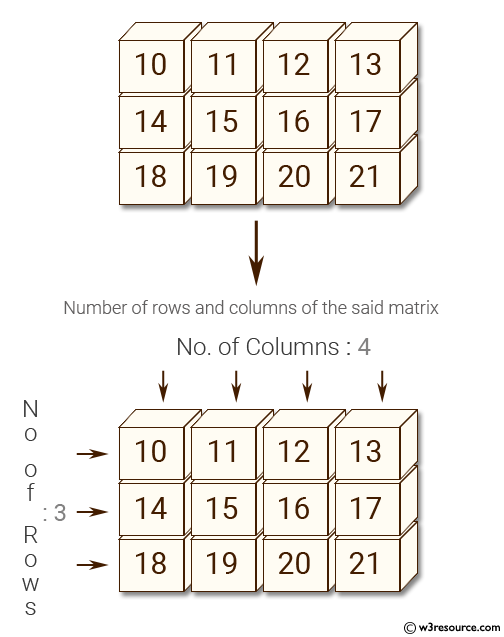﻿ NumPy: Find the number of rows and columns of a given matrix - w3resource# NumPy: Find the number of rows and columns of a given matrix

## NumPy: Basic Exercise-26 with Solution

Write a NumPy program to find the number of rows and columns of a given matrix.

Sample Solution :

Python Code :

``````import numpy as np
m= np.arange(10,22).reshape((3, 4))
print("Original matrix:")
print(m)
print("Number of rows and columns of the said matrix:")
print(m.shape)
``````

Sample Output:

```Original matrix:
[[10 11 12 13]
[14 15 16 17]
[18 19 20 21]]
Number of rows and columns of the said matrix:
(3, 4)
```

Pictorial Presentation:Python Code Editor:

Have another way to solve this solution? Contribute your code (and comments) through Disqus.

What is the difficulty level of this exercise?

Test your Python skills with w3resource's quiz

﻿

## Python: Tips of the Day

Checks if the given number falls within the given range.

Example:

```def tips_range(n, start, end = 0):
return start <= n <= end if end >= start else end <= n <= start
print(tips_range(2, 4, 6))
print(tips_range(4, 8))
print(tips_range(1, 3, 5))
print(tips_range(1, 3))
```

Output:

```False
True
False
True
```